Next: Electric scalar potential? Up: Time-dependent Maxwell's equations Previous: Introduction

The history of humankind's development of physics can be thought of as the history of the synthesis of ideas. Physicists keep finding that apparently disparate phenomena can be understood as different aspects of some more fundamental phenomenon. This process has continued until today all physical phenomena can be described in terms of three fundamental forces: gravity, the electroweak force, and the strong force. One of the main goals of modern physics is to find some way of combining these three forces so that all of physics can be described in terms of a single unified force. This, essentially, is the purpose of string theory.

The first great synthesis of ideas in physics took place in 1666 when Issac Newton realised that the force which causes apples to fall downwards is the same as the force which maintains the planets in elliptical orbits around the Sun. The second great synthesis, which we are about to study in more detail, took place in 1830 when Michael Faraday discovered that electricity and magnetism are two aspects of the same thing, usually referred to as electromagnetism. The third great synthesis, which we shall discuss presently, took place in 1873 when James Clerk Maxwell demonstrated that light and electromagnetism are intimately related. The last (but, hopefully, not the final) great synthesis took place in 1967 when Steve Weinberg and Abdus Salam showed that the electromagnetic force and the weak nuclear force (i.e., the one which is responsible for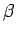decays) can be combined to give the electroweak force. Unfortunately, Weinberg's work lies well beyond the scope of this lecture course.

Let us now consider Faraday's experiments, having put them in their proper historical context. Prior to 1830, the only known way to make an electric current flow through a conducting wire was to connect the ends of the wire to the positive and negative terminals of a battery. We measure a battery's ability to push current down a wire in terms of its voltage, by which we mean the voltage difference between its positive and negative terminals. What does voltage correspond to in physics? Well, volts are the units used to measure electric scalar potential, so when we talk about a 6V battery, what we are really saying is that the difference in electric scalar potential between its positive and negative terminals is six volts. This insight allows us to write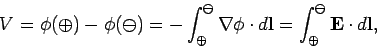(370)

whereis the battery voltage,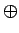denotes the positive terminal,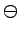the negative terminal, and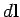is an element of length along the wire. Of course, the above equation is a direct consequence of. Clearly, a voltage difference between two ends of a wire attached to a battery implies the presence of an electric field which pushes charges through the wire. This field is directed from the positive terminal of the battery to the negative terminal, and is, therefore, such as to force electrons to flow through the wire from the negative to the positive terminal. As expected, this means that a net positive current flows from the positive to the negative terminal. The fact that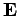is a conservative field ensures that the voltage differenceis independent of the path of the wire. In other words, two different wires attached to the same battery develop identical voltage differences.

Let us now consider a closed loop of wire (with no battery). The voltage around such a loop, which is sometimes called the electromotive force or e.m.f., is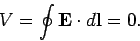(371)

This is a direct consequence of the field equation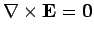. So, sinceis a conservative field then the electromotive force around a closed loop of wire is automatically zero, and no current flows around the wire. This all seems to make sense. However, Michael Faraday is about to throw a spanner in our works! He discovered in 1830 that a changing magnetic field can cause a current to flow around a closed loop of wire (in the absence of a battery). Well, if current flows through a wire then there must be an electromotive force. So,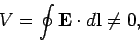(372)

which immediately implies thatis not a conservative field, and that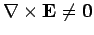. Clearly, we are going to have to modify some of our ideas regarding electric fields.

Faraday continued his experiments and found that another way of generating an electromotive force around a loop of wire is to keep the magnetic field constant and move the loop. Eventually, Faraday was able to formulate a law which accounted for all of his experiments. The e.m.f. generated around a loop of wire in a magnetic field is proportional to the rate of change of the flux of the magnetic field through the loop. So, if the loop is denoted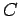, andis some surface attached to the loop, then Faraday's experiments can be summed up by writing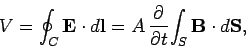(373)

whereis a constant of proportionality. Thus, the changing flux of the magnetic field through the loop creates an electric field directed around the loop. This process is know as magnetic induction.

S.I. units have been carefully chosen so as to make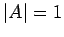in the above equation. The only thing we now have to decide is whether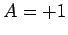or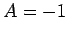. In other words, which way around the loop does the induced e.m.f. want to drive the current? We possess a general principle which allows us to decide questions like this. It is called LeChatelier's principle. According to Le Chatelier's principle, every change generates a reaction which tries to minimize the change. Essentially, this means that the Universe is stable to small perturbations. When this principle is applied to the special case of magnetic induction, it is usually called Lenz's law. According to Lenz's law, the current induced around a closed loop is always such that the magnetic field it produces tries to counteract the change in magnetic flux which generates the electromotive force. From Fig. 34, it is clear that if the magnetic field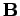is increasing and the current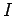circulates clockwise (as seen from above) then it generates a field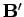which opposes the increase in magnetic flux through the loop, in accordance with Lenz's law. The direction of the current is opposite to the sense of the current loop(assuming that the flux ofthrough the loop is positive), so this implies thatin Eq. (373). Thus, Faraday's law takes the form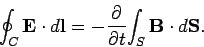(374)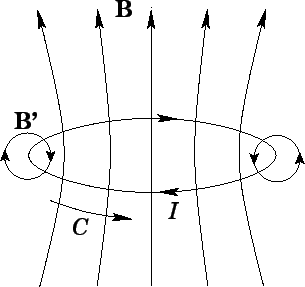Experimentally, Faraday's law is found to correctly predict the e.m.f. (i.e.,) generated in any wire loop, irrespective of the position or shape of the loop. It is reasonable to assume that the same e.m.f. would be generated in the absence of the wire (of course, no current would flow in this case). Thus, Eq. (374) is valid for any closed loop. If Faraday's law is to make any sense then it must also be true for any surfaceattached to the loop. Clearly, if the flux of the magnetic field through the loop depends on the surface upon which it is evaluated then Faraday's law is going to predict different e.m.f.s for different surfaces. Since there is no preferred surface for a general non-coplanar loop, this would not make very much sense. The condition for the flux of the magnetic field,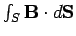, to depend only on the loopto which the surfaceis attached, and not on the nature of the surface itself, is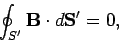(375)

for any closed surface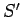.

Faraday's law, Eq. (374), can be converted into a field equation using Stokes' theorem. We obtain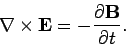(376)

This is the final Maxwell equation. It describes how a changing magnetic field can generate, or induce, an electric field. Gauss' theorem applied to Eq. (375) yields the familiar field equation(377)

This ensures that the magnetic flux through a loop is a well-defined quantity.

The divergence of Eq. (376) yields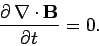(378)

Thus, the field equation (376) actually demands that the divergence of the magnetic field be constant in time for self-consistency (this means that the flux of the magnetic field through a loop need not be a well-defined quantity, as long as its time derivative is well-defined). However, a constant non-solenoidal magnetic field can only be generated by magnetic monopoles, and magnetic monopoles do not exist (as far as we are aware). Hence,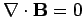. The absence of magnetic monopoles is an observational fact: it cannot be predicted by any theory. If magnetic monopoles were discovered tomorrow this would not cause physicists any problems. We know how to generalize Maxwell's equations to include both magnetic monopoles and currents of magnetic monopoles. In this generalized formalism, Maxwell's equations are completely symmetric with respect to electric and magnetic fields, and. However, an extra term (involving the current of magnetic monopoles) must be added to the right-hand side of Eq. (376) in order to make it self-consistent.Next: Electric scalar potential? Up: Time-dependent Maxwell's equations Previous: Introduction
Richard Fitzpatrick 2006-02-02